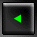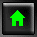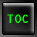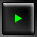##Appendix C Binary and Hexadecimal

You learned the fundamentals of arithmetic so long ago, it is hard to imagine what it would be like without that knowledge. When you look at the number 145 you instantly see "one hundred and forty-five" without much reflection.

Understanding binary and hexadecimal requires that you re-examine the number 145 and see it not as a number, but as a code for a number.

Start small: Examine the relationship between the number three and "3." The numeral 3 is a squiggle on a piece of paper; the number three is an idea. The numeral is used to represent the number.

The distinction can be made clear by realizing that three, 3, |||, III, and *** all can be used to represent the same idea of three.

In base 10 (decimal) math you use the numerals 0, 1, 2, 3, 4, 5, 6, 7, 8, 9 to represent all numbers. How is the number ten represented?

One can imagine that we would have evolved a strategy of using the letter A to represent ten; or we might have used IIIIIIIIII to represent that idea. The Romans used X. The Arabic system, which we use, makes use of position in conjunction with numerals to represent values. The first (right-most) column is used for "ones," and the next column is used for tens. Thus, the number fifteen is represented as 15 (read "one, five"); that is, 1 ten and 5 ones.

Certain rules emerge, from which some generalizations can be made:

1. Base 10 uses the digits 0-9.

2. The columns are powers of ten: 1s, 10s, 100s, and so on.

3.
If the third column is 100, the largest number you can make with two columns is 99. More generally, with n columns you can represent 0 to (10n-1). Thus, with 3 columns you can represent 0 to (103-1) or 0-999.

### Other Bases

It is not a coincidence that we use base 10; we have 10 fingers. One can imagine a different base, however. Using the rules found in base 10, you can describe base 8:

1. The digits used in base 8 are 0-7.

2.
The columns are powers of 8: 1s, 8s, 64, and so on.
3. With n columns you can represent 0 to 8n-1.

To distinguish numbers written in each base, write the base as a subscript next to the number. The number fifteen in base 10 would be written as 1510 and read as "one, five, base ten."

Thus, to represent the number 1510 in base 8 you would write 178. This is read "one, seven, base eight." Note that it can also be read "fifteen" as that is the number it continues to represent.

Why 17? The 1 means 1 eight, and the 7 means 7 ones. One eight plus seven ones equals fifteen. Consider fifteen asterisks:

```
*****     *****
```
```
*****
```

The natural tendency is to make two groups, a group of ten asterisks and another of five. This would be represented in decimal as 15 (1 ten and 5 ones). You can also group the asterisks as

```
****          *******
```
```
****
```

That is, eight asterisks and seven. That would be represented in base eight as 178. That is, one eight and seven ones.

### Around the Bases

You can represent the number fifteen in base ten as 15, in base nine as 169, in base 8 as 178, in base 7 as 217. Why 217? In base 7 there is no numeral 8. In order to represent fifteen, you will need two sevens and one 1.

How do you generalize the process? To convert a base ten number to base 7, think about the columns: in base 7 they are ones, sevens, forty-nines, three-hundred forty-threes, and so on. Why these columns? They represent 70, 71, 72, 74 and so forth. Create a table for yourself:
 4 3 2 1 73 72 71 70 343 49 7 1
The first row represents the column number. The second row represents the power of 7. The third row represents the decimal value of each number in that row.

To convert from a decimal value to base 7, here is the procedure: Examine the number and decide which column to use first. If the number is 200, for example, you know that column 4 (343) is 0, and you don't have to worry about it.

To find out how many 49s there are, divide 200 by 49. The answer is 4, so put 4 in column 3 and examine the remainder: 4. There are no 7s in 4, so put a zero in the sevens column. There are 4 ones in 4, so put a 4 in the 1s column. The answer is 4047.

To convert the number 968 to base 6:
 5 4 3 2 1 64 63 62 61 60 1296 216 36 6 1
There are no 1296s in 968, so column 5 has 0. Dividing 968 by 216 yields 4 with a remainder of 104. Column 4 is 4. Dividing 104 by 36 yields 2 with a remainder of 32. Column 3 is 2. Dividing 32 by 6 yields 5 with a remainder of 2. The answer therefore is 42526.
 5 4 3 2 1 64 63 62 61 60 1296 216 36 6 1 0 4 2 5 2
There is a shortcut when converting from one base to another base (such as 6) to base 10. You can multiply:
 4 * 216 = 864 2 * 36 = 72 5 * 6 = 30 2 * 1 = 2 968

#### Binary

Base 2 is the ultimate extension of this idea. There are only two digits: 0 and 1. The columns are:
 Col: 8 7 6 5 4 3 2 1 Power: 27 26 25 24 23 22 21 20 Value: 128 64 32 16 8 4 2 1
To convert the number 88 to base 2, you follow the same procedure: There are no 128s, so column 8 is 0.

There is one 64 in 88, so column 7 is 1 and 24 is the remainder. There are no 32s in 24 so column 6 is 0.

There is one 16 in 24 so column 5 is 1. The remainder is 8. There is one 8 in 8, and so column 4 is 1. There is no remainder, so the rest of the columns are 0.
 0 1 0 1 1 0 0 0
To test this answer, convert it back:

```
1 * 64 =  64
0 * 32 =   0
1 * 16 =  16
1 *  8 =   8
0 *  4 =   0
0 *  2 =   0
0 *  1 =   0                88
```

#### Why Base 2?

The power of base 2 is that it corresponds so cleanly to what a computer needs to represent. Computers do not really know anything at all about letters, numerals, instructions, or programs. At their core they are just circuitry, and at a given juncture there either is a lot of power or there is very little.

To keep the logic clean, engineers do not treat this as a relative scale (a little power, some power, more power, lots of power, tons of power), but rather as a binary scale ("enough power" or "not enough power"). Rather than saying "enough" or "not enough," they simplify it to "yes" or "no." Yes or no, or true or false, can be represented as 1 or 0. By convention, 1 means true or Yes, but that is just a convention; it could just as easily have meant false or no.

Once you make this great leap of intuition, the power of binary becomes clear: With 1s and 0s you can represent the fundamental truth of every circuit (there is power or there isn't). All a computer ever knows is, "Is you is, or is you ain't?" Is you is = 1; is you ain't = 0.

#### Bits, Bytes, and Nybbles

Once the decision is made to represent truth and falsehood with 1s and 0s, binary digits (or bits) become very important. Since early computers could send 8 bits at a time, it was natural to start writing code using 8-bit numbers--called bytes.

NOTE: Half a byte (4 bits) is called a nybble!

With 8 binary digits you can represent up to 256 different values. Why? Examine the columns: If all 8 bits are set (1), the value is 255. If none is set (all the bits are clear or zero) the value is 0. 0-255 is 256 possible states.

#### Whats a KB?

It turns out that 210 (1,024) is roughly equal to 103 (1,000). This coincidence was too good to miss, so computer scientists started referring to 210 bytes as 1KB or 1 kilobyte, based on the scientific prefix of kilo for thousand.

Similarly, 1024 * 1024 (1,048,576) is close enough to one million to receive the designation 1MB or 1 megabyte, and 1,024 megabytes is called 1 gigabyte (giga implies thousand-million or billion).

#### Binary Numbers

Computers use patterns of 1s and 0s to encode everything they do. Machine instructions are encoded as a series of 1s and 0s and interpreted by the fundamental circuitry. Arbitrary sets of 1s and 0s can be translated back into numbers by computer scientists, but it would be a mistake to think that these numbers have intrinsic meaning.

For example, the Intel 80x6 chip set interprets the bit pattern 1001 0101 as an instruction. You certainly can translate this into decimal (149), but that number per se has no meaning.

Sometimes the numbers are instructions, sometimes they are values, and sometimes they are codes. One important standardized code set is ASCII. In ASCII every letter and punctuation is given a 7-digit binary representation. For example, the lowercase letter "a" is represented by 0110 0001. This is not a number, although you can translate it to the number 97 (64 + 32 + 1). It is in this sense that people say that the letter "a" is represented by 97 in ASCII; but the truth is that the binary representation of 97, 01100001, is the encoding of the letter "a," and the decimal value 97 is a human convenience.

Because binary numbers are difficult to read, a simpler way to represent the same values is sought. Translating from binary to base 10 involves a fair bit of manipulation of numbers; but it turns out that translating from base 2 to base 16 is very simple, because there is a very good shortcut.

To understand this, you must first understand base 16, which is known as hexadecimal. In base 16 there are sixteen numerals: 0, 1, 2, 3, 4, 5, 6, 7, 8, 9, A, B, C, D, E, and F. The last six are arbitrary; the letters A-F were chosen because they are easy to represent on a keyboard. The columns in hexadecimal are
 4 3 2 1 163 162 161 160 4096 256 16 1
To translate from hexadecimal to decimal, you can multiply. Thus, the number F8C represents:

```
F * 256 = 15 * 256 = 3840
8 * 16 =		128
C * 1 = 12 * 1 =	12
3980
```

Translating the number FC to binary is best done by translating first to base 10, and then to binary:

```
F * 16 = 15 * 16 =  240
C * 1 = 12 * 1 =     12
252
```

Converting 25210 to binary requires the chart:

```
Col:       9     8    7   6    5   4    3    2    1
Power:     28   27   26   25   24  23   22   21   20
Value:   256   128   64  32   16   8    4    2    1

There are no 256s.
1 128 leaves 124
1 64 leaves 60
1 32 leaves 28
1 16 leaves 12
1 8 leaves 4
1 4 leaves 0
0
0
1    1    1    1    1    1    0    0
```

Thus, the answer in binary is 1111 1100.

Now, it turns out that if you treat this binary number as two sets of 4 digits, you can do a magical transformation.

The right set is 1100. In decimal that is 12, or in hexadecimal it is C.

The left set is 1111, which in base 10 is 15, or in hex is F.

Thus, you have:

```1111 1100
F     C
```

Putting the two hex numbers together is FC, which is the real value of 1111 1100. This shortcut always works. You can take any binary number of any length, and reduce it to sets of 4, translate each set of four to hex, and put the hex numbers together to get the result in hex. Here's a much larger number:

```1011 0001 1101 0111
```

The columns are 1, 2, 4, 8, 16, 32, 64, 128, 256, 512, 1024, 2048, 4096, 8192, 16384, and 32768.

```1 x 1 =		1
1 x 2=		2
1 x 4 =		4
0 x 8 =		0

1 x 16 =		16
0 x 32 =		0
1 x 64 =		64
1 x 128 =		128

1 x 256 =		256
0 x 512 =		0
0 x 1024 =		0
0 x 2048 =		0

1 x 4096 =		4,096
1 x 8192 =		8,192
0 x 16384 =	0
1 x 32768 =	32,768
Total:		45,527```

```65535    4096    256    16    1
```

There are no 65,536s in 45,527 so the first column is 4096. There are 11 4096s (45,056), with a remainder of 471. There is one 256 in 471 with a remainder of 215. There are 13 16s (208) in 215 with a remainder of 7. Thus, the hexadecimal number is B1D7.

Checking the math:

```B (11) * 4096 =	45,056
1 * 256 =		256
D (13) * 16 =	 208
7 * 1 =		7
Total		45,527
```
```The shortcut version would be to take the original binary number, 1011000111010111,
and break it into groups of 4: 1011 0001 1101 0111. Each of the four then
is evaluated as a hexadecimal number: ```

 ``` 1011 =``` `1 x 1 =` ``` 1 ``` ``` 1 x 2 = ``` ``` 2 ``` ``` 0 x 4 = ``` ``` 0 ``` ``` 1 x 8 = ``` ``` 8 ``` ``` Total ``` ``` 11 ``` ``` Hex: ``` ``` B ``` ``` 0001 =``` ``` 1 x 1 = ``` ``` 1 ``` ``` 0 x 2 = ``` ``` 0 ``` ``` 0 x 4 = ``` ``` 0 ``` ``` 0 * 8 = ``` ``` 0 ``` ``` Total ``` ``` 1 ``` ``` Hex: ``` ``` 1 ``` ``` 1101 =``` ``` 1 x 1 = ``` ``` 1 ``` ``` 0 x 2 = ``` ``` 0 ``` ``` 1 x 4 = ``` ``` 4 ``` ``` 1 x 8 = ``` ``` 8 ``` ``` Total ``` ``` 13 ``` ``` Hex = ``` ``` D ``` ``` 0111 =``` ``` 1 x 1 = ``` ``` 1 ``` ``` 1 x 2 = ``` ``` 2 ``` ``` 1 x 4 = ``` ``` 4 ``` ``` 0 x 8 = ``` ``` 0 ``` ``` Total ``` ``` 7 ``` ``` Hex: ``` ``` 7 ```
Total Hex: B1D7